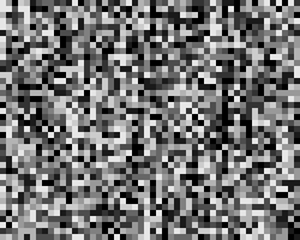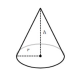## Mindhack – Mental math pseudo-random number generators

Human minds are great for many things, but picking random numbers is not one of them1. At one point, these sorts of cognitive biases were used to support arguments for determinism, to the point where some experimental psychologists even undertook to train subjects to produce statistically random-looking output2. Determinism is beyond the scope of this post, but in keeping with the spirit of the last, we'll be looking at simple mathematical tricks to generate pseudo-random numbers.

DISCLAIMER: These techniques are not suitable for practical applications, and especially not any serious cryptography. I am not an expert in the field, and have just been playing with these ideas in my spare time.

### Background

Computer programs often need quasi-random numbers for everything from scientific simulations to cryptography; unfortunately, true randomness is difficult to extract, and usually limited in quantity. However, not all applications require the same level of randomness: cryptography needs to protect against a dedicated human adversary, and so has the greatest need for numbers being actually random3, while scientific simulations only require that the numbers look indistinguishable from random ones by certain statistical tests, but need lots of them as quickly as possible, even if they aren't quite as "random".

To fill those niches, mathematicians and computer scientists have developed a wide range of different pseudo random number generators (PRNG), each with their pluses and minuses. What exactly are PRNGs? Simply put, they're sets of mathematical rules for deterministically producing random-looking output. Because they are deterministic, they require an initial seed number, and always produce the same output for the same seed4. However, to most humans who aren't statisticians or cryptanalysts, they're sufficiently good.

Here, we present three simple PRNG algorithms, implementations of the Lehmer PRNG5, that you can carry around in the back of your head for when you need to wow an audience with your ability to randomise, . For the record, I am not to be held liable if you lose all of your friends, stutter at interviews, or get hit by a bus because you're too busy updating your internal PRNG.

### Lehmer PRNG

First some (skippable) maths: given a large prime$p$, a multiplier$m$, and a seed number$x_0$, a Lehmer PRNG can be defined by the recursive function$x_{n+1} = m \cdot x_n \mod p$ 6. By a judicious choice of$m$, the series$\{x_i\}$ does not repeat for a long time, ideally going through all of the integers from$1$ to$p-1$ 7. The output stream is then some function of the current state$x_i$. For computer programs$p, m$ are usually chosen for ease of binary computation. Furthermore, computers can handle far bigger numbers than most of us mere humans can—the historically popular choice8$2^{31} -1$ is a lovely number, but I'd hate to be trying to divide by it in my head. Luckily, there are lots of primes, and good choices make it far easier to do modular arithmetic in decimal notation, reducing everything to basic addition/multiplication on the digits. Furthermore, instead of taking the bit parity or least/most significant bit, we instead use the unit's digit for the output stream.

One pair choice is$p=59, m=6$ 9. Because$60 \equiv 1 \mod 59$, each iteration is a simple matter of multiplying the ones digit by 6 and adding the tens digit. To illustrate, a sequence starting from 17 would continue 43, 22, 14, 25, 32, 15, 31, 9, 54, 29,..., and taking just the unit's digit, that turns into 3, 2, 4, 5, 2, 5, 1, 9, 4, 9, which looks pretty random. Naturally, since the modulus is only 59, the sequence repeats itself every 58 iterations. Also, the numbers 9 and 0 are slightly less well-represented in the stream than the others. In most everyday contexts neither would be a problem—unless your friends have a habit of forcing you to spit out hundreds of random numbers and then running statistical analysis10, and if that's the case, I suggest you find new friends. However, there are other, slightly harder to compute pairs if you want a bit more.

Next up is$p=101, m=50$. Because$p=101$, this construction has the advantage that the sequence goes from 1 to 100, making the distribution of the output stream uniform over 1 to 10 and providing a longer period. The choice of multiplier also simplifies computation: if the current state$x_i$ is even, the next number$x_{i+1} = 101 - \frac{x_i}{2}$, and if odd,$x_{i+1} = 50 - \frac{x_i-1}{2}$. Not as nice as the previous example, but still not bad.

If you have far too much time on your hands, you might consider an even larger prime to increase the period. For example, the pair$p=1999, m=20$. Unlike the previous two examples, the period of this Lehmer PRNG isn't actually the full 1998, but rather "only" 999. While another choice of multiplier would give a better answer, 20 has the advantage of making computing far simpler. Besides, it's fairly likely that you'll make a math error before getting to 999 that'll put you in the other group of 999 anyways. For the calculation, first break up$x_i = 100 a_i + b_i$, where $a_i, b_i$ are two digit numbers. Then$x_{i+1} = 20\cdot b_i + a_i$. Or equivalently, take the smallest two digits, multiply them by 2 and shift them to the left one place, and then add the larger two digits. Some mental gymnastics required, but still do-able.Visual analysis of (p=1999, m=20). Bitmap image generated by linking pixel brightness to the values of output stream. 9 corresponds to white, 0 to black, and shades of grey to everything in between.

And of course, there are an infinite number of primes, so if none of the above suits your fancy, pick another. For ease of computation, I've found it best to choose primes close to$k \cdot 10^n$, because with those you can simplify the arithmetic as we've done above. However, the sky's the limit.

No, I'm not talking metaphorically (though the referenced article does propose a good idea). Now that you have an internal PRNG, you'll have to start worrying about the initial values. Luckily, unlike a computer, you have the entire world around you, and there are lots of options. Maybe look at the seconds hand on a clock, count the number of clouds in the sky, or just throw some dice. Just add the values to the current state, and voila, you've jumped to a completely different section in the output stream. This is especially suggested if you use the (1999,20) Lehmer PRNG, because otherwise you'll always be stuck on only 999 of the possible states.

Indeed, for those of you who aren't happy with the idea of trying to forever keep a 2-4 digit number in your head, you can just use these methods as transformations of common numbers, a substitution cipher for numbers to make them seem more random. Just think of the date/time whenever someone asks you for a random number, use it as your current state, and give the next iteration to as many digits as required. Won't quite be random, but it's probably still more than enough. Happy number crunching!

As an aside, some parapsychologists (both cranks and more reputable researchers, like the Global Consciousness Project) believe that it's possible for people to mentally influence random numbers through quantum effects. I'm not going give my opinion on the validity of those claims, but if you use these PRNGs, because it's completely deterministic, you're safe from their influence!

1. Wagenaar, W. A. Generation of random sequences by human subjects: A critical survey of literature. Psychological Bulletin, 77, 65-72 (1972) (URI).
2. Neuringer, A. Can People Behave "Randomly?": The Role of Feedback. Journal of Experimental Psychology, 115, 62-75 (1986).
3. For crypto, either true random numbers from an external source or specialised cryptographically secure PRNGs are needed. Cryptographically secure PRNG algorithms tend to be far too complicated for mental math, though I was really intrigued by Blum Blum Shub.
4. That PRNGs always produce the same output for the same seed is exploited by stream ciphers for encryption.
5. I had wanted to do a Linear Feedback Shift Register as well, but unfortunately those don't work well in base 10, since 10 isn't prime.
6. I'm simplifying the maths deliberately; Lehmer PRNGs are actually a slightly larger class of methods.
7.$m$ needs to have high multiplicative order modulo$p$, and$\{x_i\} = \{i\in \mathbb{R}: 1\le i \le p-1\}$ when$p$ is a primitive root.
8. Park, S.K. & Miller, K.W. Random number generators: good ones are hard to find. Comm. of the ACM 31, 1192-1201 (1998) (URI).
9. Thanks to an online post a while back by the late Professor Marsaglia. Unfortunately, I can't seem to readily find the reference anymore. Edit: found by courtesy of Josh Jordan.
10. It's actually extremely easy to cryptanalyse Lehmer PRNGs, given the right tools, which is why they shouldn't be used for anything secure.

1.gwern says:
2. [...] a piece of paper and some time, this mathy mindhack can continuously generate quasi-random [...]

3.Thomas Dybdahl Ahle says:

I just realized you didn't take "mod p^6" and didn't take "m=6^9". Superscripts and footnotes don't go well together.

Other than that, great article!

•William says:

Ach. That's definitely confusing; will need to look into other footnote plugins, I guess. Glad you enjoyed!

4.Pavel says:

Minor bug: that should be m*x[n] mod p, not m*x mod p.

•William says:

Oops. Corrected. Thanks!

5.Josh Jordan says:

•William says:

Thanks for the find! I've updated the reference and given you credit for finding it 🙂

6. Maternal says:

paginas.seccionamarilla.com.mx

die ewige Zier

7. perder barriga homem Magro

die ewige Zier